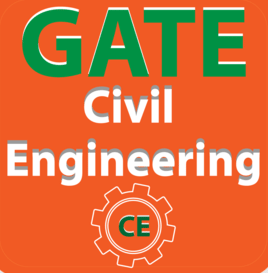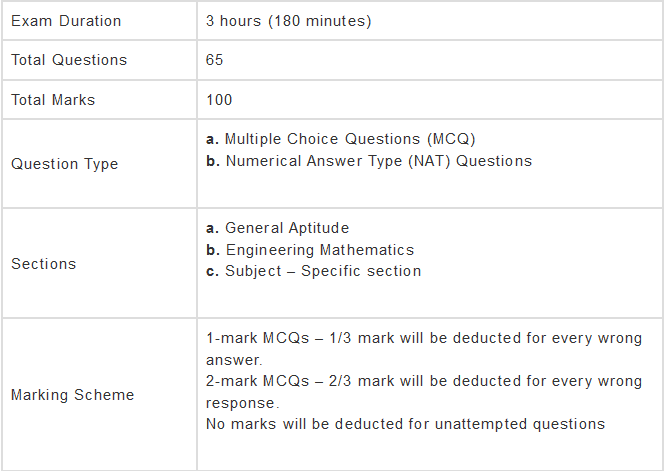# Syllabus - Civil Engineering, GATE - Practice Notes - GATE

## GATE: Syllabus - Civil Engineering, GATE - Practice Notes - GATE

The document Syllabus - Civil Engineering, GATE - Practice Notes - GATE is a part of GATE category.
All you need of GATE at this link: GATEBefore getting into the details of GATE Civil Engineering Syllabus, let’s have an overview of GATE Exam Pattern for Civil Engineering:The three sections of the GATE paper are:
(i) General Aptitude
(ii) Engineering Mathematics
(iii) Subject-Specific Section

Let us now look into the detailed GATE syllabus for Civil Engineering.
Syllabus for General Aptitude (GA) (Common to all Papers)

• Verbal Ability: English grammar, sentence completion, verbal analogies, word groups, instructions, critical reasoning and verbal deduction.
• Numerical Ability: Numerical computation, numerical estimation, numerical reasoning and data interpretation.

Civil Engineering

1. Engineering Mathematics

• Linear Algebra: Matrix Algebra, Systems of linear equations, Eigenvalues, Eigenvectors.
• Calculus: Mean value theorems, Theorems of integral calculus, Evaluation of definite and improper integrals, Partial Derivatives, Maxima and minima, Multiple integrals, Fourier series, Vector identities, Directional derivatives, Line integral, Surface integral, Volume integral, Stokes’s theorem, Gauss’s theorem, Green’s theorem.
• Differential Equations: First order equations (linear and nonlinear), Higher order linear differential equations with constant coefficients, Method of variation of parameters, Cauchy’s equation, Euler’s equation, Initial and boundary value problems, Partial Differential Equations, Method of separation of variables.
• Complex Variables: Analytic functions, Cauchy’s integral theorem, Cauchy’s integral formula, Taylor series, Laurent series, Residue theorem, Solution integrals.
• Probability and Statistics: Sampling theorems, Conditional probability, Mean, Median, Mode, Standard Deviation, Random variables, Discrete and Continuous distributions, Poisson distribution, Normal distribution, Binomial distribution, Correlation analysis, Regression analysis.
• Numerical Methods: Solutions of nonlinear algebraic equations, Single and Multi‐step methods for differential equations.

2. Structural Engineering

2.1 Engineering Mechanics

• System of forces, free-body diagrams, equilibrium equations.
• Internal forces in structures.
• Friction and its applications.
• Kinematics of point mass and rigid body.
• Centre of mass.
• Euler’s equations of motion.
• Impulse-momentum.
• Energy methods.
• Principles of virtual work.

2.2 Solid Mechanics

• Bending moment and shear force in statically determinate beams.
• Simple stress and strain relationships.
• Theories of failures.
• Simple bending theory, flexural and shear stresses, Shear Center.
• Uniform torsion, buckling of column, combined and direct bending stresses.

2.3 Structural Analysis

• Statically determinate and indeterminate structures by force/ energy methods.
• Method of superposition.
• Analysis of trusses, arches, beams, cables and frames.
• Displacement methods: Slope deflection and moment distribution methods.
• Influence lines.
• Stiffness and flexibility methods of structural analysis.

2.4 Construction Materials and Management

• Construction Materials: Structural steel – composition, material properties and behaviour.
• Concrete – constituents, mix design, short-term and long-term properties.
• Bricks and mortar.
• Timber Bitumen.

2.5 Concrete Structures

• Working stress, Limit state, and Ultimate load design concepts.
• Design of beams, slabs, columns.
• Bond and development length.
• Prestressed concrete.
• Analysis of beam sections at transfer and service loads.

2.6 Steel Structures

• Working Stress and Limit state design concepts.
• Design of tension and compression members, beams and beam-columns, column bases.
• Connections - simple and eccentric, beam-column connections, plate girders, and trusses.
• Plastic analysis of beams and frames.

3. Geotechnical Engineering

3.1 Soil Mechanics

• Origin of soils, soil structure, and fabric.
• Three-phase system and phase relationships, index properties.
• Unified and Indian standard soil classification system.
• Permeability - one-dimensional flow, Darcy’s law.
• Seepage through soils - two-dimensional flow, flow nets, uplift pressure, piping.
• The principle of effective stress, capillarity, seepage force and quicksand condition.
• Compaction in laboratory and field conditions.
• One dimensional consolidation, the time rate of consolidation.
• Mohr’s circle, stress paths, effective and total shear strength parameters, characteristics of clays and sand.

3.2 Foundation Engineering

• Sub-surface investigations - scope, drilling boreholes, sampling, plate load test, standard penetration and cone penetration tests.
• Earth pressure theories - Rankine and Coulomb.
• Stability of slopes - finite and infinite slopes, a method of slices and Bishop’s method.
• Stress distribution in soils - Boussinesq’s and Westergaard’s theories, pressure bulbs.
• Shallow foundations - Terzaghi’s and Meyerhoff’s bearing capacity theories, an effect of the water table.
• Combined footing and raft foundation.
• Contact pressure.
• Settlement analysis in sands and clays.
• Deep foundations - types of piles, dynamic and static formulae, load capacity of piles in sands and clays, pile load test, negative skin friction.

4. Water Resources Engineering

4.1 Fluid Mechanics

• Properties of fluids, fluid statics.
• Continuity, momentum, energy and corresponding equations.
• Potential flow, applications of momentum and energy equations.
• Laminar and turbulent flow.
• Flow in pipes, pipe networks.
• Concept of boundary layer and its growth.

4.2 Hydraulics

• Forces on immersed bodies.
• Flow measurement in channels and pipes.
• Dimensional analysis and hydraulic similitude.
• Kinematics of flow, velocity triangles.
• Basics of hydraulic machines, specific speed of pumps and turbines.
• Channel Hydraulics - Energy-depth relationships, specific energy, critical flow, slope profile, hydraulic jump, uniform flow and gradually varied flow.

4.3 Hydrology

• Hydrologic cycle
• Precipitation
• Evaporation
• Eevapo-transpiration
• Watershed
• Infiltration
• Unit hydrographs
• Hydrograph analysis.
• Flood estimation and routing.
• Reservoir capacity, reservoir and channel routing.
• Surface run-off models.
• Groundwater hydrology - steady state well hydraulics and aquifer.
• Application of Darcy’s law.

4.4 Irrigation

• Duty, delta, estimation of evapotranspiration.
• Crop water requirements.
• Design of lined and unlined canals, head works, gravity dams and spillways.
• Design of weirs on permeable foundation.
• Types of irrigation systems, irrigation methods.
• Waterlogging and drainage.
• Canal regulatory works, cross-drainage structures, outlets and escapes.

5. Environmental Engineering

5.1 Water and Waste Water

• Quality standards, basic unit processes, and operations for water treatment.
• Drinking water standards, water requirements, basic unit operations and unit processes for surface water treatment, distribution of water. Sewage and sewerage treatment, quantity and characteristics of wastewater. Primary, secondary and tertiary treatment of wastewater, effluent discharge standards.
• Domestic wastewater treatment, the quantity of characteristics of domestic wastewater, primary and secondary treatment.
• Unit operations and unit processes of domestic wastewater, sludge disposal.

5.2 Air Pollution

• Types of pollutants, their sources, and impacts.
• Air pollution meteorology.
• Air pollution control.
• Air quality standards and limits.

5.3 Municipal Solid Wastes

• Characteristics
• Generation
• Collection and transportation of solid wastes, engineered systems for solid waste management (reuse/ recycle, energy recovery, treatment and disposal).

5.4 Noise Pollution

• Impacts of noise.
• Permissible limits of noise pollution.
• Measurement of noise and control of noise pollution.

6. Transportation Engineering

6.1 Transportation Infrastructure

• Highway alignment and engineering surveys.
• The geometric design of highways - cross-sectional elements, sight distances, horizontal and vertical alignments.
• The geometric design of railway track.
• Airport runway length, taxiway, and exit taxiway design.

6.2 Highway Pavements

• Highway materials - desirable properties and quality control tests.
• Design of bituminous paving mixes.
• Design factors for flexible and rigid pavements.
• Design of flexible pavement using IRC: 37-2012.
• Design of rigid pavements using IRC: 58-2011.
• Distresses in concrete pavements.

6.3 Traffic Engineering

• Traffic studies on flow, speed, travel time - delay and O-D stud.
• PCU, peak hour factor, a parking study, accident study and analysis, statistical analysis of traffic data.
• Microscopic and Macroscopic parameters of traffic flow, fundamental relationships.
• Control devices, signal design by Webster’s method.
• Types of intersections and channelization.
• Highway capacity and level of service of rural highways and urban roads.

7. Geomatics Engineering

• Principles of surveying.
• Maps - scale, co-ordinate system.
• Distance and angle measurement - Levelling and trigonometric levelling.
• Traversing and triangulation survey.
• Total station.
• Horizontal and vertical curves.
• Photogrammetry - scale, flying height.
• Remote sensing - basics, platform and sensors, visual image interpretation.
• Basics of Geographical information system (GIS) and Geographical Positioning System (GPS).
The document Syllabus - Civil Engineering, GATE - Practice Notes - GATE is a part of GATE category.
All you need of GATE at this link: GATEUse Code STAYHOME200 and get INR 200 additional OFF

Track your progress, build streaks, highlight & save important lessons and more!

,

,

,

,

,

,

,

,

,

,

,

,

,

,

,

,

,

,

,

,

,

,

,

,

;